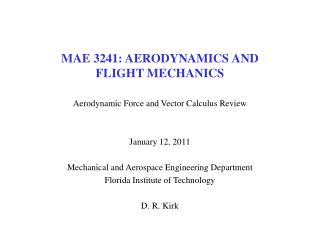# MAE 3241: AERODYNAMICS AND FLIGHT MECHANICS - PowerPoint PPT PresentationDownload PresentationMAE 3241: AERODYNAMICS AND FLIGHT MECHANICS

MAE 3241: AERODYNAMICS AND FLIGHT MECHANICSDownload Presentation## MAE 3241: AERODYNAMICS AND FLIGHT MECHANICS

- - - - - - - - - - - - - - - - - - - - - - - - - - - E N D - - - - - - - - - - - - - - - - - - - - - - - - - - -
##### Presentation Transcript

1. MAE 3241: AERODYNAMICS ANDFLIGHT MECHANICS Aerodynamic Force and Vector Calculus Review January 12, 2011 Mechanical and Aerospace Engineering Department Florida Institute of Technology D. R. Kirk

2. READING ASSIGNMENT • Fundamentals of Aerodynamics, 5th edition • Chapter 1 • All sections except: 1.7 and 1.9 • Chapter 2 • All sections except: 2.7

3. WHAT CREATES AERODYNAMIC FORCES? • Aerodynamic forces exerted by airflow comes from only two sources • Pressure, p, distribution on surface • Acts normal to surface • Shear stress, tw, (friction) on surface • Acts tangentially to surface • Pressure and shear are in units of force per unit area (N/m2) • Net unbalance creates an aerodynamic force “No matter how complex the flow field, and no matter how complex the shape of the body, the only way nature has of communicating an aerodynamic force to a solid object or surface is through the pressure and shear stress distributions that exist on the surface.” “The pressure and shear stress distributions are the two hands of nature that reach out and grab the body, exerting a force on the body – the aerodynamic force”

4. VECTOR CALCULUS REVIEW (SECTION 2.2) • Natural and convenient representation to explain motion of fluids in 3D space • Expression of field quantities • Position • Velocity • Acceleration • Vorticity • Forces • Directly transfer to scalar forms to solve problems • Advantage of vector calculus/algebra: • Definition and theorems are independent of coordinate system • In scalar form expression are different between cartesian, cylindrical, spherical, etc. coordinate systems

5. VECTOR CALCULUS REVIEW • The curl of the gradient of a scalar function is zero • The divergence of the curl of a vector is zero • If the curl of the velocity field is zero • Flow is irrotational • Velocity can be written as the gradient of a scalar function, f • If the divergence of the velocity field is zero • Flow is incompressible • If both are true • Laplace equation

6. SUMMARY OF VECTOR INTEGRALS • Gradient Theorem • Vector equation involving a scalar function, a • Limits of integration such that surface encloses the volume • n points normal outward • Divergence (Gauss’) Theorem • Scalar equation • Stokes Theorem • Direction of n is given by right hand rule

7. SCRAMJET PROPULSION: X-43 MACH 10!

8. EXAMPLE: SHOCK ENHANCED MIXING • Rayleigh scattering image of flow field development following M=1.146 shock passage over a cylinder of helium embedded in air • Difficult to explain with velocity/pressure field description • Vorticity field useful for insight • Shockwave moving left to right in pictures • Interacting with helium cylinder in pictures (a) – (c) shockwave